# Three-sigma rule

(diff) ← Older revision | Latest revision (diff) | Newer revision → (diff)

A rule of thumb, according to which, in certain problems in probability theory and mathematical statistics, an event is considered to be practically impossible if it lies in the region of values of the normal distribution of a random variable at a distance from its mathematical expectation of more than three times the standard deviation.

Letbe a normally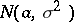distributed random variable. For any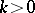,where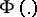is the distribution function of the standard normal law; whence, in particular, for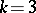it follows that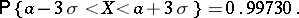The latter equation means that the values ofcan differ from its expectationby a quantity exceedingon the average in not more than 3 times in a thousand trials. This circumstance is sometimes used by an experimenter in certain problems of probability theory and mathematical statistics, by assuming that the event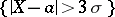is practically impossible and, consequently, the event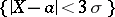is practically certain. In this case one says that the experimenter has applied the "three-sigma" rule.

How to Cite This Entry:
Three-sigma rule. Encyclopedia of Mathematics. URL: http://encyclopediaofmath.org/index.php?title=Three-sigma_rule&oldid=17366
This article was adapted from an original article by M.S. Nikulin (originator), which appeared in Encyclopedia of Mathematics - ISBN 1402006098. See original article